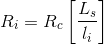### 5. Deflection Angle Method

The traditional method of staking a spiral is by measuring a deflection angles at the TS and chords between curve points. In Figure E-10, the process to lay out the first two points after the TS are:

• Instrument at TS, sighting PI.
• To stake point i
• Rotate to deflection angle of i, ai.
• Measure distance LTSI-i
• Stake point j
• Rotate to deflection angle of j, aj.
• Measure distance Li-j

The arc distances between the points (ie, Staj - Stai) are used as chords. While this does introduce some error, because of the spiral's geometry, the error is relatively small.Figure E-10 Deflection Angle Method

General calculations are based on these spiral properties for any point referenced to the flat end:

• Radius is inversely proportional to distance along the spiral.
• Spiral angle is proportional to the square of the distance
• Deflection angle is closely proportional to the square of the distance
• Tangent offset is closely proportional to the cube of the distance.Figure E-11 Deflection Angle Geometry

For any spiral point i:Equation E-16 Arc distanceEquation E-17 Spiral radiusEquation E-18 Spiral angle, radiansEquation E-19 Spiral angle, degreesEquation E-20 Deflection angle, degrees

Although not needed for the traditional deflection angle method, the tangent distance and offset to a curve point, Figure E-12, can be computed using Equations E-21 and E-22.Equation E-21Equation E-22
Figure E-12
Tangent Distance and Offset

Tangent distances (xi) and offsets (yi) can be used to compute radial chords from the TS to the respective curve point, Figure E-13 and Equation E-23.Figure E-13 Radial ChordEquation E-23

As with the point-to-point condition, generally the arc distances can be used as radial chord distances. We see this shortly in an example.